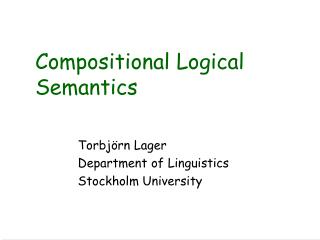Download PresentationCompositional Logical Semantics

Compositional Logical Semantics - PowerPoint PPT Presentation

Compositional Logical Semantics. Torbjörn Lager Department of Linguistics Stockholm University. Logical Semantics Example. John laughed laughed'(j) Nobody laughed  x[laughed'(x)] But this is just translation! What's semantic about that?. What Is the Name of This Game?.I am the owner, or an agent authorized to act on behalf of the owner, of the copyrighted work described.
Download PresentationCompositional Logical Semantics

Download Policy: Content on the Website is provided to you AS IS for your information and personal use and may not be sold / licensed / shared on other websites without getting consent from its author.While downloading, if for some reason you are not able to download a presentation, the publisher may have deleted the file from their server.

- - - - - - - - - - - - - - - - - - - - - - - - - - E N D - - - - - - - - - - - - - - - - - - - - - - - - - -
Presentation Transcript
1. Compositional Logical Semantics Torbjörn Lager Department of Linguistics Stockholm University

2. Logical Semantics Example • John laughed • laughed'(j) • Nobody laughed • x[laughed'(x)] • But this is just translation! What's semantic about that? NLP1 - Torbjörn Lager

3. What Is the Name of This Game? • Truth conditional semantics • Model theoretic semantics • Logical semantics • Formal semantics • Compositional semantics • Syntax-driven semantic analysis • Compositional, logical, truth conditional, model theoretic semantics .... NLP1 - Torbjörn Lager

4. We use language to talk about the world Meaning = Truth conditions Examples: "John whistles" is true iff John whistles "John visslar" is true iff John whistles "Ogul fautu seq" is true iff... Truth Conditional Semantics Natural language The outside world NLP1 - Torbjörn Lager

5. We don't know what the world is really like, so let's talk about a model of the world instead Such a model does (usually) consists of individuals, sets of individuals, functions and relations. i.e the sort of things set theory talks about Truth becomes truth relative to a model Model Theoretic Semantics Natural language Model The outside world NLP1 - Torbjörn Lager

6. The Compositionality Principle: The meaning of the whole is a function of the meaning of the parts and the mode of combining them. The meaning of a complex expression is uniquely determined by the meaning of its constituents and the syntactic construction used to combine them. Compositional Semantics Natural language Model The World NLP1 - Torbjörn Lager

7. A simple model M: Domain: {John, Mary, Paul} Interpretation: Names: "John" refers to John , "Mary" refers to Mary, etc. Verbs: "whistles" refers to {John, Paul} Example "John whistles" is true in M iff the individual in M referred to as "John" is an element in the set of individuals that "whistles" refer to. Truth Conditional, Model Theoretic and Compositional Semantics Combined Richard Montague (1970): "I reject the contention that an important theoretical difference exists between formal and natural languages" NLP1 - Torbjörn Lager

8. Account for the meanings of natural language utterances by translating them into another language. It could be any language, but only if this language has a formal semantics are we done. Translational Semantics Natural language Logical Form Language Model The World NLP1 - Torbjörn Lager

9. Have same truth conditions Grammar and Logical Form • S -> NP VP[S] = [VP]([NP]) • NP -> john[NP] = j • VP -> whistles[VP] = x[whistles'(x)] • [john whistles] = whistles'(j) cf. The principle of compositionality NLP1 - Torbjörn Lager

10. Beta Reduction (Lambda Conversion) • [S] = [VP]([NP]) • [NP] = j • [VP] = x[whistles'(x)] • Beta reduction rule: u()   where every occurrence of u in  is replaced by • x[whistles'(x)](j) application • whistles'(j) reduction NLP1 - Torbjörn Lager

11. Grammar and Logical Form • S -> NP VP[S] = [NP]([VP]) • NP -> john[NP] = P[P(j)] • VP -> whistles[VP] = x[whistles'(x)] • [john whistles] = whistles'(j) NLP1 - Torbjörn Lager

12. From Logical Form to Truth Conditions • whistles'(j) is true iffthe individual (in the model) denoted by 'j' has the property denoted by 'whistles' • cf. "John whistles" is true iff John whistles NLP1 - Torbjörn Lager

13. Beta Reduction (Lambda Conversion) • [S] = [NP]([VP]) • [NP] = P[P(j)] • [VP] = x[whistles'(x)] • Beta reduction rule: u()   where every occurrence of u in  is replaced by • P[P(j)](x[whistles'(x)]) application • x[whistles'(x)](j) reduction • whistles'(j) reduction NLP1 - Torbjörn Lager

14. A Larger Example • S -> NP VP [S] = [NP]([VP]) • NP -> DET N [NP] = [DET]([N]) • DET -> every[DET] = Q[P[z[Q(z)  P(z)]]] • N -> man [N] = x[man'(x)] • VP -> whistles[VP] = x[whistles'(x)] • [every man whistles} = z[man'(z)  whistles'(z)] NLP1 - Torbjörn Lager

15. A Larger Example (cont'd) • [S] = [NP]([VP]) • [NP] = [DET]([N]) • [DET] = Q[P[z[Q(z)  P(z)]]] • [N] = x[man'(x)] • [VP] = x[whistles'(x)] • Q[P[z[Q(z)  P(z)]]](x[man'(x)]) application • P[z[x[man'(x)](z)  P(z)]] reduction • P[z[man'(z)  P(z)]] reduction • P[z[man'(z)  P(z)]](x[whistles'(x)]) application • z[man'(z) x[whistles'(x)](z)] reduction • z[man'(z)  whistles'(z)] reduction NLP1 - Torbjörn Lager

16. Examples in Oz • X = {fun {\$ P} {P j} end fun {\$ X} whistles(X) end} • % X gets bound to the tuple 'whistles(j)' • DetSem = fun {\$ Q} fun {\$ P} all(Z impl({Q Z} {P Z})) end end • NSem = fun {\$ X} man(X) end • VPSem = fun {\$ X} whistles(X) end • NPSem = {DetSem NSem} • SSem = {NPSem VPSem} • % X gets bound to the tuple 'all(Z impl(man(Z) whistles(Z)))' NLP1 - Torbjörn Lager